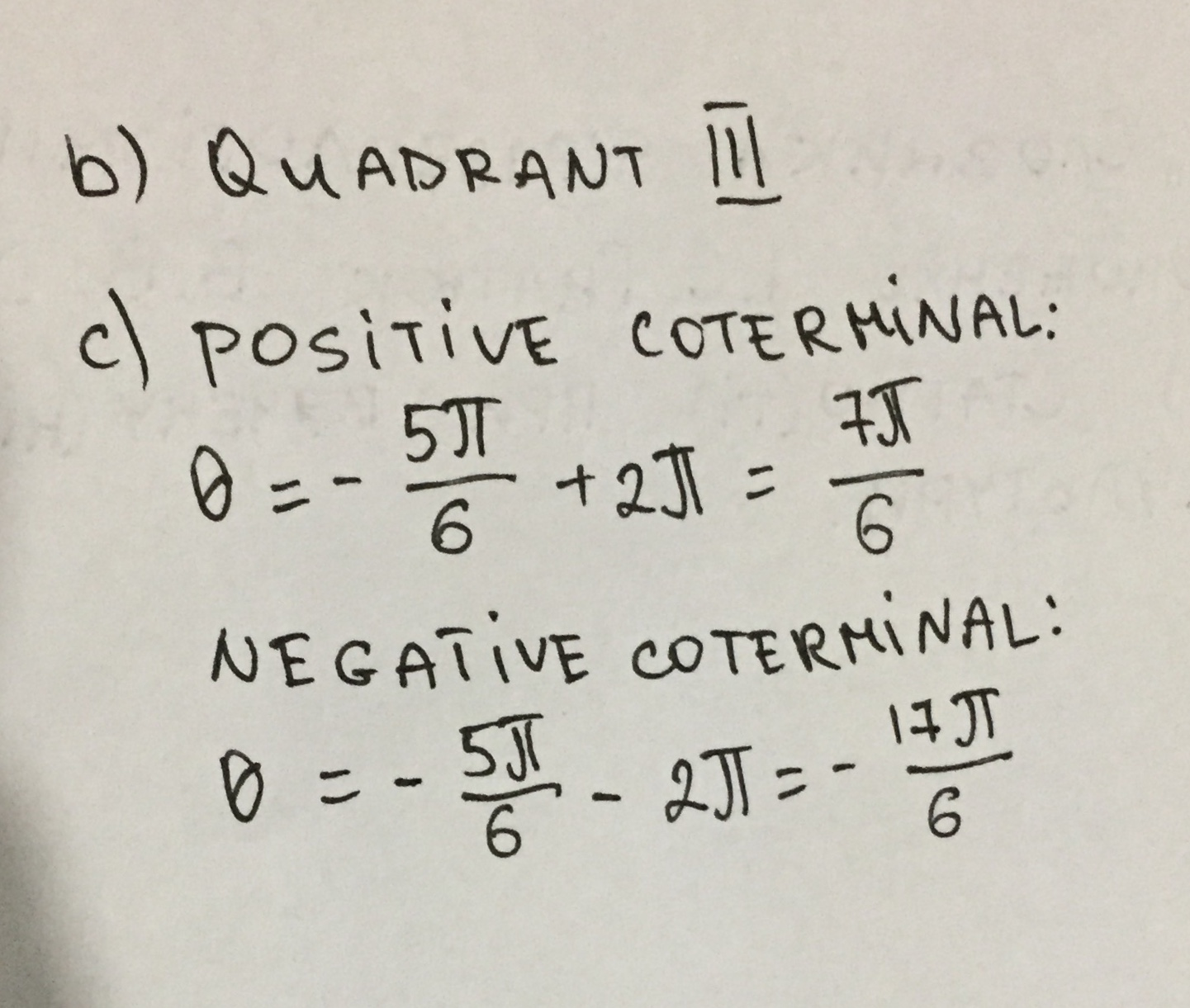Question# a) Determine the quadrant in which the angle lies, and

Negative numbers and coordinate plane
ANSWEREDa) Determine the quadrant in which the angle lies, and
(b) list one positive and one negative coterminal $$\displaystyle\angle−{5}\frac{\pi}{{6}}$$​.2021-08-18

b) We find coterminals of $$\displaystyleθ$$ by adding or subtracting integer multiples of $$\displaystyle{2}\pi$$:
$$\displaystyleθ=-{5}\frac{\pi}{{6}}+{2}\pi={7}\frac{\pi}{{6}}$$ - positive
$$\displaystyleθ=-{5}\frac{\pi}{{6}}–{2}\pi=-{17}\frac{\pi}{{6}}$$ - negative